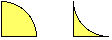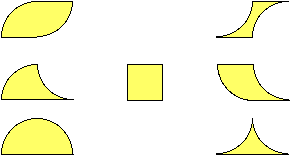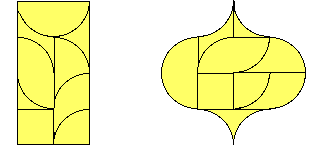https://www.mathed.page/puzzles/polyarcs/index.html

# Polyarcs

By Henri Picciotto

Consider the two pieces that are created by drawing a quarter circle with radius one, centered at a corner of a unit square, and cutting along the resulting arc:We will call these pieces monarcs.

1. What is the perimeter and area of each monarc?
2. What is the average area of the two monarcs?

Two monarcs can be put together to create diarcs in seven distinct ways. Here they are:1. What is the perimeter and area of each diarc?
2. What is the average area of the diarcs?

All the monarcs and diarcs can be combined into a 2 by 4 rectangle, or a pleasingly symmetric curvilinear figure.1. What is the area and perimeter of each figure?
2. Find all the triarcs.
3. Find interesting figures made of diarcs and triarcs.

## Discussion

1. When does the area of a polyarc figure involve pi? When does it not?
2. When does the perimeter of a polyarc figure involve pi? When does it not?
3. In #5, some students argue that since both figures consist of the same pieces, they must have the same perimeter and area. Explain why they are right or wrong.